# Iterating over rows and columns in Pandas DataFrame

Iteration is a general term for taking each item of something, one after another. Pandas DataFrame consists of rows and columns so, in order to iterate over dataframe, we have to iterate a dataframe like a dictionary. In a dictionary, we iterate over the keys of the object in the same way we have to iterate in dataframe.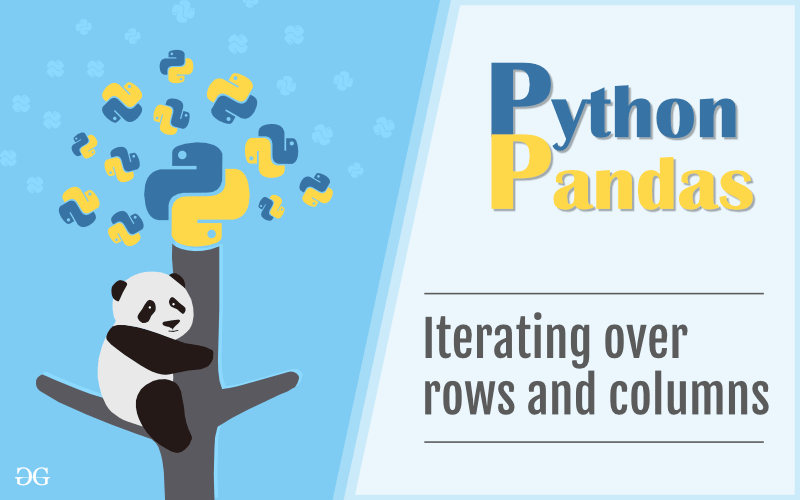In this article, we are using “`nba.csv`” file to download the CSV, click here.

In Pandas Dataframe we can iterate an element in two ways:

• Iterating over rows
• Iterating over columns

### Iterating over rows :

In order to iterate over rows, we can use three function `iteritems()`, ` iterrows()`, `itertuples() `. These three function will help in iteration over rows.

#### Iteration over rows using `iterrows()`

In order to iterate over rows, we apply a `iterrows()` function this function return each index value along with a series containing the data in each row.

Code #1:

 `# importing pandas as pd ` `import` `pandas as pd ` `  `  `# dictionary of lists ` `dict` `=` `{``'name'``:[``"aparna"``, ``"pankaj"``, ``"sudhir"``, ``"Geeku"``], ` `        ``'degree'``: [``"MBA"``, ``"BCA"``, ``"M.Tech"``, ``"MBA"``], ` `        ``'score'``:[``90``, ``40``, ``80``, ``98``]} ` ` `  `# creating a dataframe from a dictionary  ` `df ``=` `pd.DataFrame(``dict``) ` ` `  `print``(df) `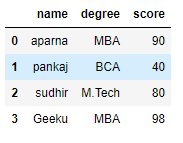Now we apply `iterrows()` function in order to get a each element of rows.

 `# importing pandas as pd ` `import` `pandas as pd ` `  `  `# dictionary of lists ` `dict` `=` `{``'name'``:[``"aparna"``, ``"pankaj"``, ``"sudhir"``, ``"Geeku"``], ` `        ``'degree'``: [``"MBA"``, ``"BCA"``, ``"M.Tech"``, ``"MBA"``], ` `        ``'score'``:[``90``, ``40``, ``80``, ``98``]} ` ` `  `# creating a dataframe from a dictionary  ` `df ``=` `pd.DataFrame(``dict``) ` ` `  `# iterating over rows using iterrows() function  ` `for` `i, j ``in` `df.iterrows(): ` `    ``print``(i, j) ` `    ``print``() `

Output: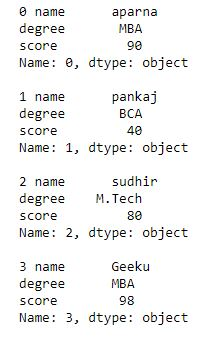Code #2:

 `# importing pandas module  ` `import` `pandas as pd  ` `    `  `# making data frame from csv file  ` `data ``=` `pd.read_csv(``"nba.csv"``)  ` ` `  `# for data visulaization we filter first 3 datasets ` `data.head(``3``) `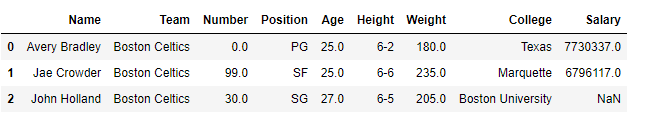Now we apply a iterrows to get each element of rows in dataframe

 `# importing pandas module  ` `import` `pandas as pd  ` `    `  `# making data frame from csv file  ` `data ``=` `pd.read_csv(``"nba.csv"``)  ` ` `  `for` `i, j ``in` `data.iterrows(): ` `    ``print``(i, j) ` `    ``print``() `

Output: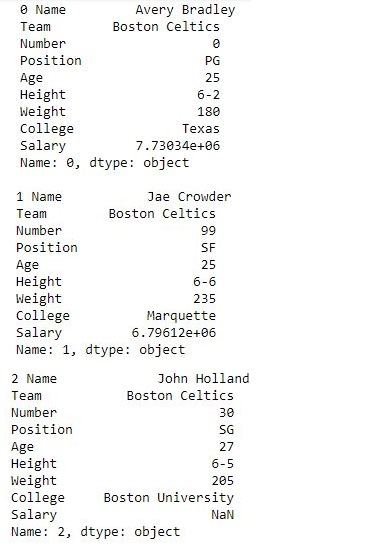#### Iteration over rows using `iteritems()`

In order to iterate over rows, we use `iteritems()` function this function iterates over each column as key, value pair with label as key and column value as a Series object.

Code #1:

 `# importing pandas as pd ` `import` `pandas as pd ` `  `  `# dictionary of lists ` `dict` `=` `{``'name'``:[``"aparna"``, ``"pankaj"``, ``"sudhir"``, ``"Geeku"``], ` `        ``'degree'``: [``"MBA"``, ``"BCA"``, ``"M.Tech"``, ``"MBA"``], ` `        ``'score'``:[``90``, ``40``, ``80``, ``98``]} ` ` `  `# creating a dataframe from a dictionary  ` `df ``=` `pd.DataFrame(``dict``) ` ` `  `print``(df) `Now we apply a `iteritems()` function in order to retrieve an rows of dataframe.

 `# importing pandas as pd ` `import` `pandas as pd ` `  `  `# dictionary of lists ` `dict` `=` `{``'name'``:[``"aparna"``, ``"pankaj"``, ``"sudhir"``, ``"Geeku"``], ` `        ``'degree'``: [``"MBA"``, ``"BCA"``, ``"M.Tech"``, ``"MBA"``], ` `        ``'score'``:[``90``, ``40``, ``80``, ``98``]} ` ` `  `# craeting a dataframe from a dictionary  ` `df ``=` `pd.DataFrame(``dict``) ` ` `  `# using iteritems() function to retrieve rows ` `for` `key, value ``in` `df.iteritems(): ` `    ``print``(key, value) ` `    ``print``() `

Output: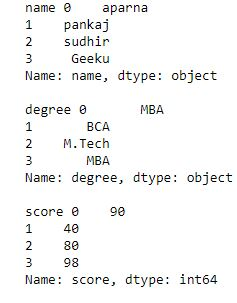Code #2:

 `# importing pandas module  ` `import` `pandas as pd  ` `    `  `# making data frame from csv file  ` `data ``=` `pd.read_csv(``"nba.csv"``)  ` ` `  `# for data visualization we filter first 3 datasets ` `data.head(``3``) `

Output:Now we apply a `iteritems()` in order to retrieve rows from a dataframe

 `# importing pandas module  ` `import` `pandas as pd  ` `    `  `# making data frame from csv file  ` `data ``=` `pd.read_csv(``"nba.csv"``)  ` ` `  `for` `key, value ``in` `data.iteritems(): ` `    ``print``(key, value) ` `    ``print``() `

Output: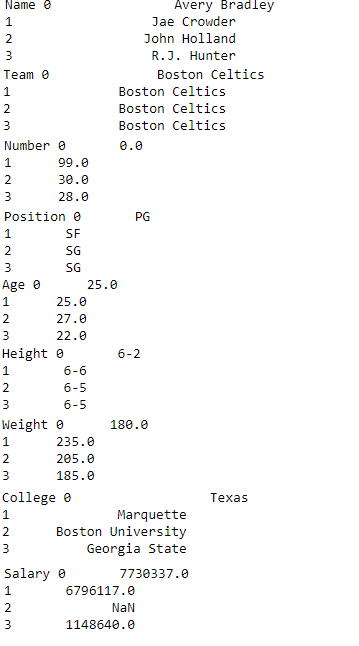#### Iteration over rows using `itertuples()`

In order to iterate over rows, we apply a function `itertuples()` this function return a tuple for each row in the DataFrame. The first element of the tuple will be the row’s corresponding index value, while the remaining values are the row values.

Code #1:

 `# importing pandas as pd ` `import` `pandas as pd ` `  `  `# dictionary of lists ` `dict` `=` `{``'name'``:[``"aparna"``, ``"pankaj"``, ``"sudhir"``, ``"Geeku"``], ` `        ``'degree'``: [``"MBA"``, ``"BCA"``, ``"M.Tech"``, ``"MBA"``], ` `        ``'score'``:[``90``, ``40``, ``80``, ``98``]} ` ` `  `# creating a dataframe from a dictionary  ` `df ``=` `pd.DataFrame(``dict``) ` ` `  `print``(df) `Now we apply a `itertuples()` function inorder to get tuple for each row

 `# importing pandas as pd ` `import` `pandas as pd ` `  `  `# dictionary of lists ` `dict` `=` `{``'name'``:[``"aparna"``, ``"pankaj"``, ``"sudhir"``, ``"Geeku"``], ` `        ``'degree'``: [``"MBA"``, ``"BCA"``, ``"M.Tech"``, ``"MBA"``], ` `        ``'score'``:[``90``, ``40``, ``80``, ``98``]} ` ` `  `# creating a dataframe from dictionary  ` `df ``=` `pd.DataFrame(``dict``) ` ` `  `# using a itertuples()  ` `for` `i ``in` `df.itertuples(): ` `    ``print``(i) `

Output: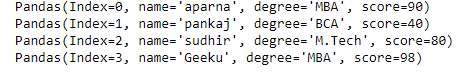Code #2:

 `# importing pandas module  ` `import` `pandas as pd  ` `    `  `# making data frame from csv file  ` `data ``=` `pd.read_csv(``"nba.csv"``)  ` ` `  `# for data visualization we filter first 3 datasets ` `data.head(``3``) `Now we apply an `itertuples()` to get atuple of each rows

 `# importing pandas module  ` `import` `pandas as pd  ` `    `  `# making data frame from csv file  ` `data ``=` `pd.read_csv(``"nba.csv"``)  ` ` `  `for` `i ``in` `data.itertuples(): ` `    ``print``(i) `

Output: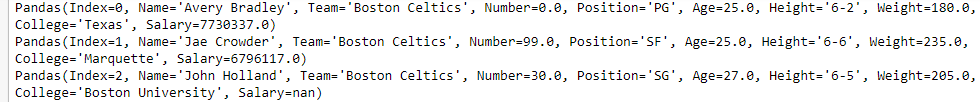### Iterating over Columns :

In order to iterate over columns, we need to create a list of dataframe columns and then iterating through that list to pull out the dataframe columns.

Code #1:

 `# importing pandas as pd ` `import` `pandas as pd ` `   `  `# dictionary of lists ` `dict` `=` `{``'name'``:[``"aparna"``, ``"pankaj"``, ``"sudhir"``, ``"Geeku"``], ` `        ``'degree'``: [``"MBA"``, ``"BCA"``, ``"M.Tech"``, ``"MBA"``], ` `        ``'score'``:[``90``, ``40``, ``80``, ``98``]} ` `  `  `# creating a dataframe from a dictionary  ` `df ``=` `pd.DataFrame(``dict``) ` ` `  `print``(df) `Now we iterate through columns in order to iterate through columns we first create a list of dataframe columns and then iterate through list.

 `# creating a list of dataframe columns ` `columns ``=` `list``(df) ` ` `  `for` `i ``in` `columns: ` ` `  `    ``# printing the third element of the column ` `    ``print` `(df[i][``2``]) `

Output: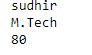Code #2:

 `# importing pandas module  ` `import` `pandas as pd  ` `    `  `# making data frame from csv file  ` `data ``=` `pd.read_csv(``"nba.csv"``)  ` ` `  `# for data visualization we filter first 3 datasets ` ` ``col ``=` `data.head(``3``) ` ` `  `col `Now we iterate over columns in CSV file in order to iterate over columns we create a list of dataframe columns and iterate over list

 `# creating a list of dataframe columns ` `clmn ``=` `list``(col) ` ` `  `for` `i ``in` `clmn: ` `    ``# printing a third element of column ` `    ``print``(col[i][``2``]) `

Output: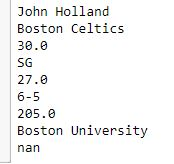My Personal Notes arrow_drop_up
Article Tags :

7

Please write to us at contribute@geeksforgeeks.org to report any issue with the above content.